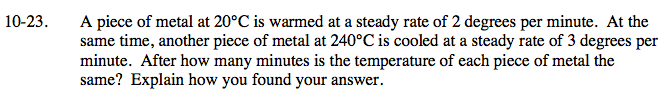### Home > CAAC > Chapter 10 > Lesson 10.1.2 > Problem10-23

10-23.Define the variables.
x = number of minutes
y = temperature of the metal

Create a system of equations to find the two unknowns.
y = 2x + 20
y = −3x + 240

x = 44 minutes